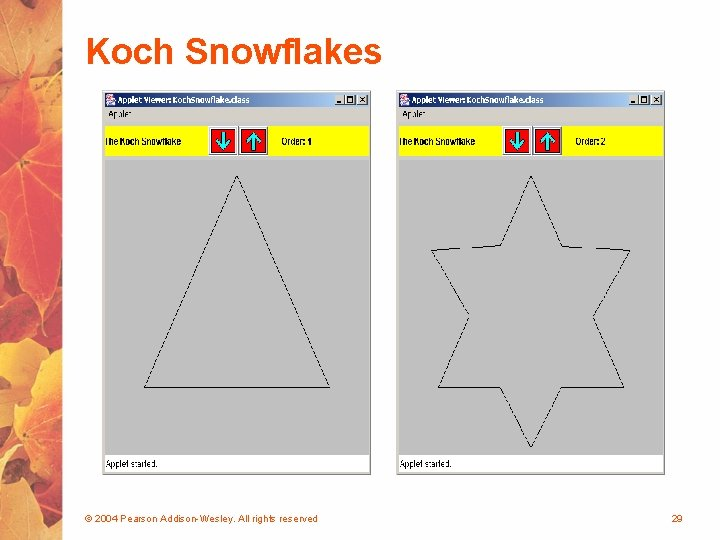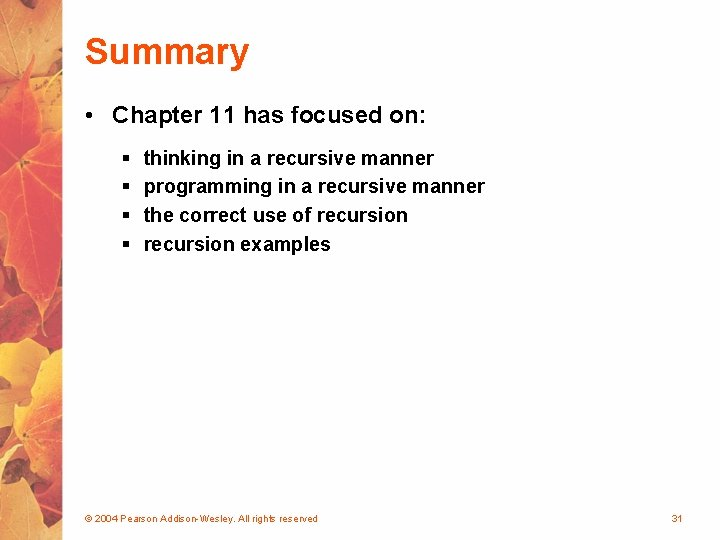# Chapter 11 Recursion Recursion Recursion is a fundamental

• Slides: 31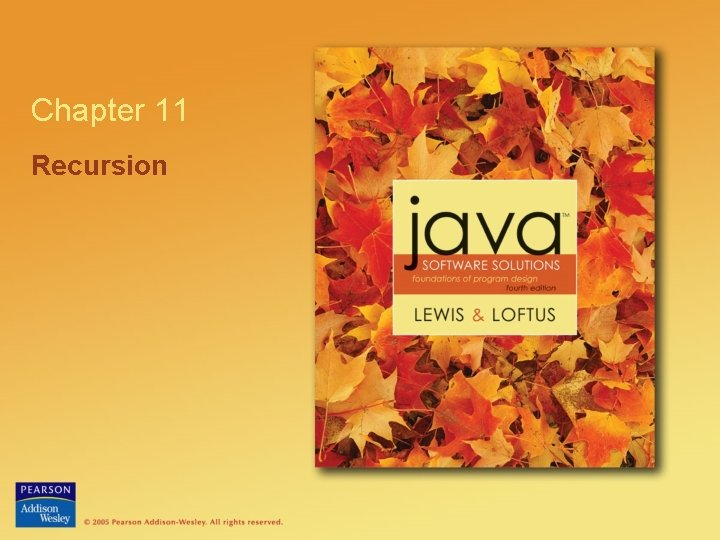Chapter 11 RecursionRecursion • Recursion is a fundamental programming technique that can provide an elegant solution certain kinds of problems • Chapter 11 focuses on: § § thinking in a recursive manner programming in a recursive manner the correct use of recursion examples © 2004 Pearson Addison-Wesley. All rights reserved 2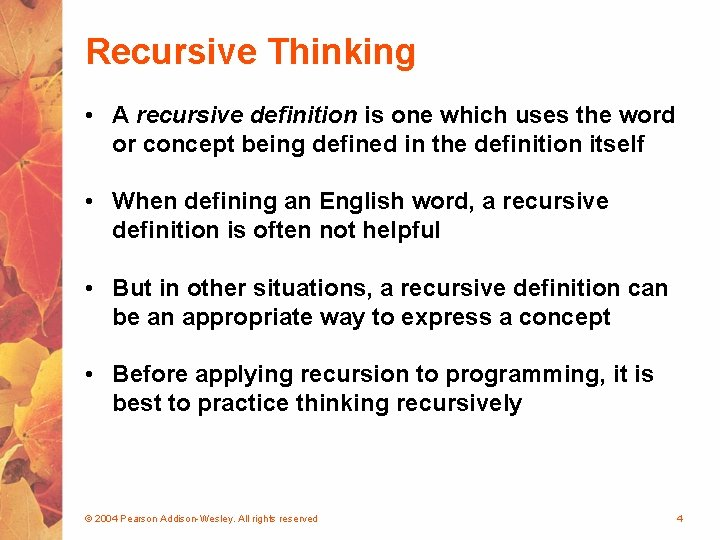Recursive Thinking • A recursive definition is one which uses the word or concept being defined in the definition itself • When defining an English word, a recursive definition is often not helpful • But in other situations, a recursive definition can be an appropriate way to express a concept • Before applying recursion to programming, it is best to practice thinking recursively © 2004 Pearson Addison-Wesley. All rights reserved 4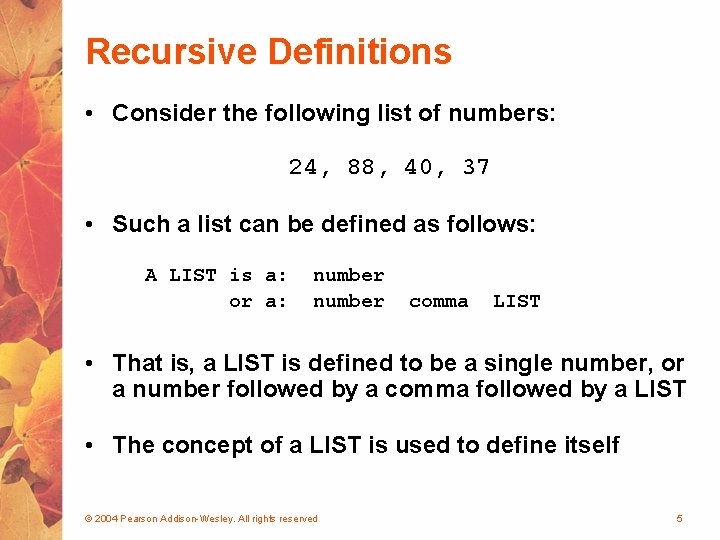Recursive Definitions • Consider the following list of numbers: 24, 88, 40, 37 • Such a list can be defined as follows: A LIST is a: or a: number comma LIST • That is, a LIST is defined to be a single number, or a number followed by a comma followed by a LIST • The concept of a LIST is used to define itself © 2004 Pearson Addison-Wesley. All rights reserved 5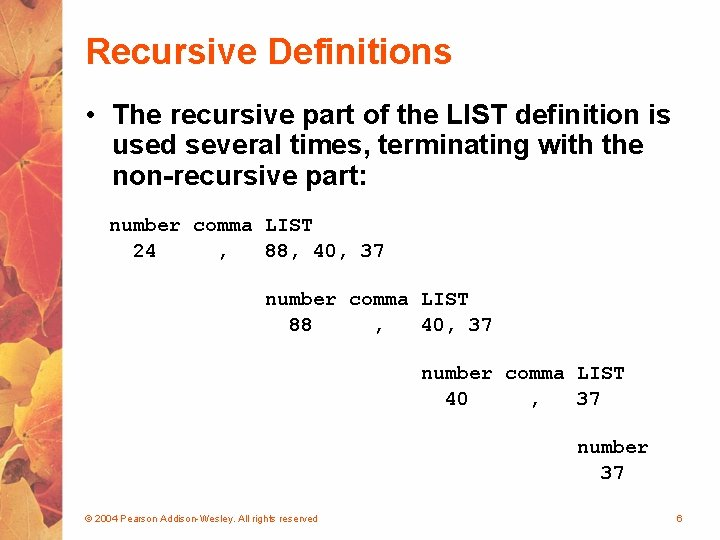Recursive Definitions • The recursive part of the LIST definition is used several times, terminating with the non-recursive part: number comma LIST 24 , 88, 40, 37 number comma LIST 88 , 40, 37 number comma LIST 40 , 37 number 37 © 2004 Pearson Addison-Wesley. All rights reserved 6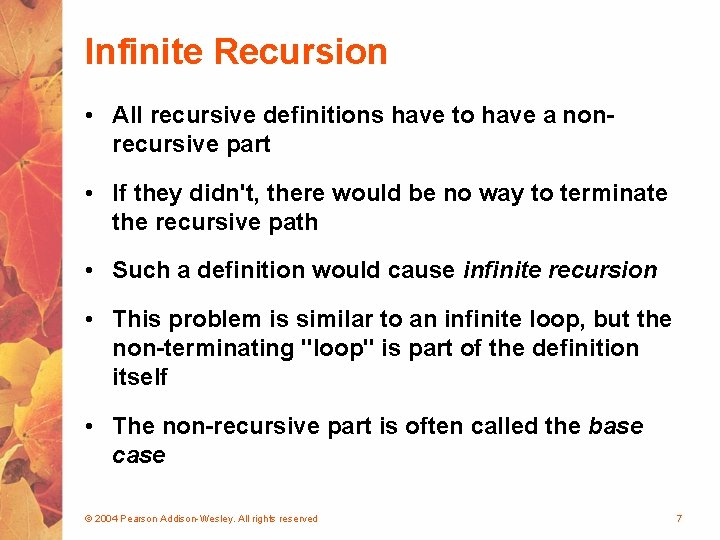Infinite Recursion • All recursive definitions have to have a nonrecursive part • If they didn't, there would be no way to terminate the recursive path • Such a definition would cause infinite recursion • This problem is similar to an infinite loop, but the non-terminating "loop" is part of the definition itself • The non-recursive part is often called the base case © 2004 Pearson Addison-Wesley. All rights reserved 7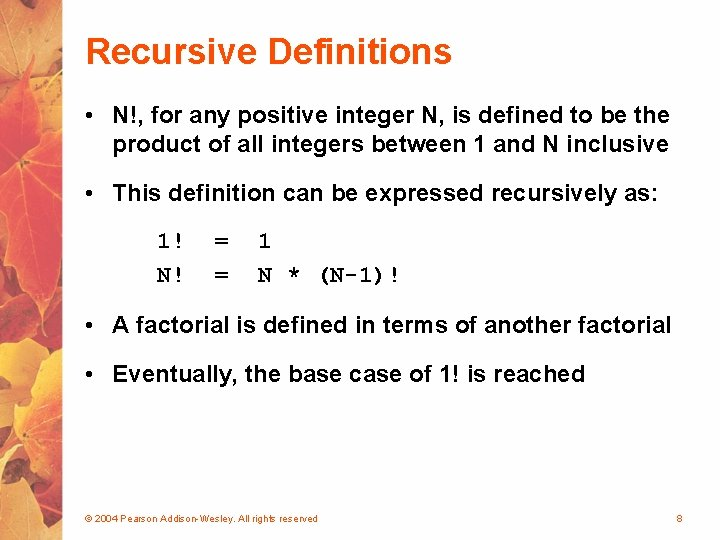Recursive Definitions • N!, for any positive integer N, is defined to be the product of all integers between 1 and N inclusive • This definition can be expressed recursively as: 1! N! = = 1 N * (N-1)! • A factorial is defined in terms of another factorial • Eventually, the base case of 1! is reached © 2004 Pearson Addison-Wesley. All rights reserved 8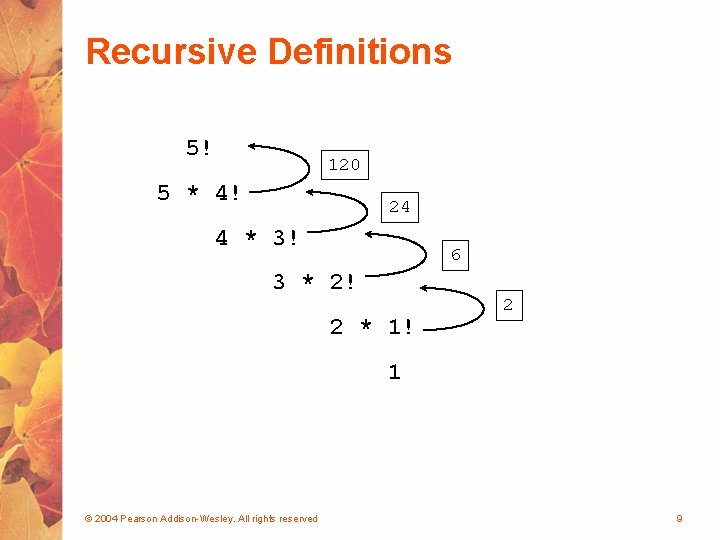Recursive Definitions 5! 120 5 * 4! 24 4 * 3! 6 3 * 2! 2 * 1! 2 1 © 2004 Pearson Addison-Wesley. All rights reserved 9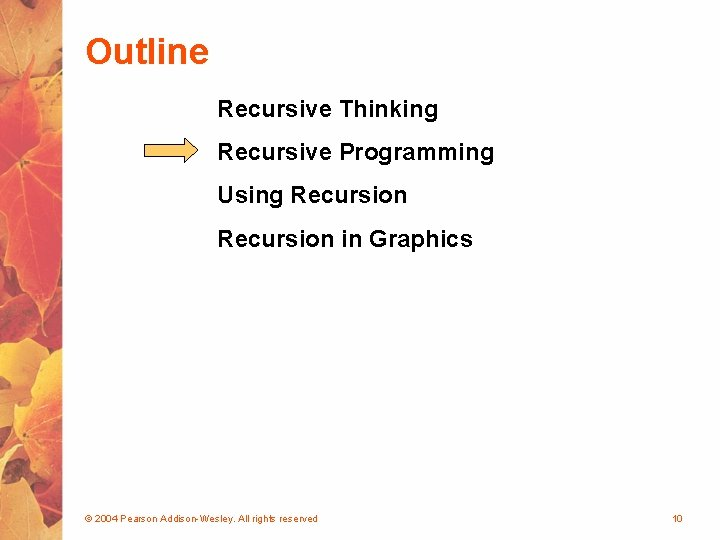Recursive Programming • A method in Java can invoke itself; if set up that way, it is called a recursive method • The code of a recursive method must be structured to handle both the base case and the recursive case • Each call to the method sets up a new execution environment, with new parameters and local variables • As with any method call, when the method completes, control returns to the method that invoked it (which may be an earlier invocation of itself) © 2004 Pearson Addison-Wesley. All rights reserved 11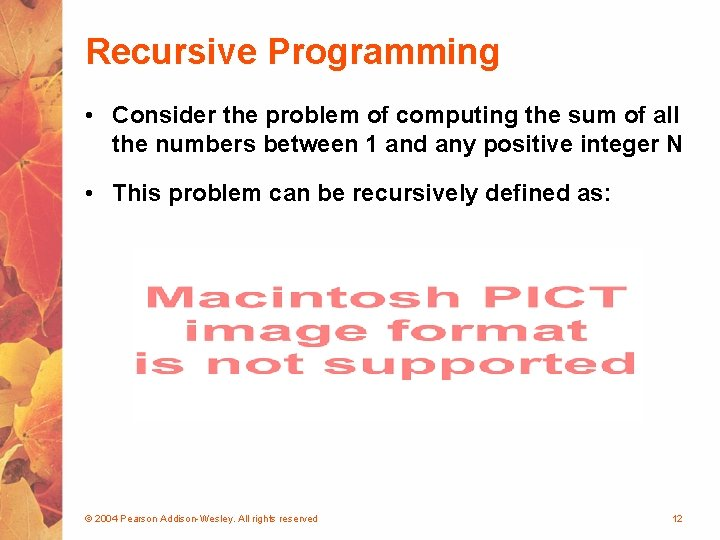Recursive Programming • Consider the problem of computing the sum of all the numbers between 1 and any positive integer N • This problem can be recursively defined as: © 2004 Pearson Addison-Wesley. All rights reserved 12Recursive Programming // This method returns the sum of 1 to num public int sum (int num) { int result; if (num == 1) result = 1; else result = num + sum (n-1); return result; } © 2004 Pearson Addison-Wesley. All rights reserved 13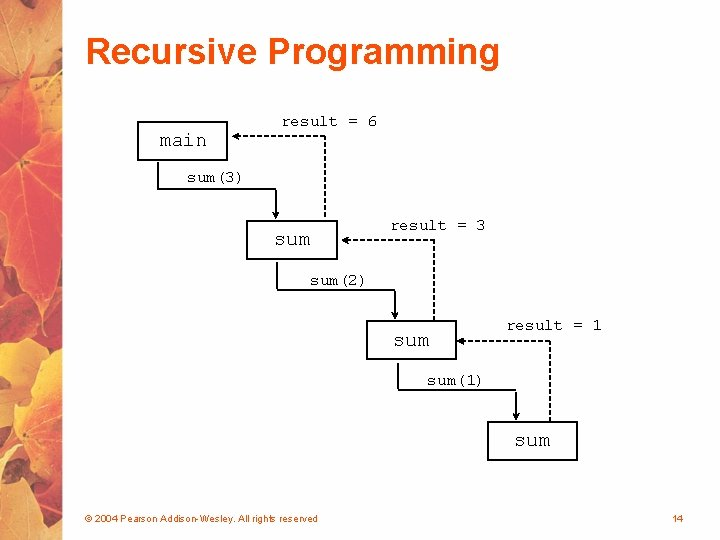Recursive Programming • Note that just because we can use recursion to solve a problem, doesn't mean we should • For instance, we usually would not use recursion to solve the sum of 1 to N problem, because the iterative version is easier to understand • However, for some problems, recursion provides an elegant solution, often cleaner than an iterative version • You must carefully decide whether recursion is the correct technique for any problem © 2004 Pearson Addison-Wesley. All rights reserved 15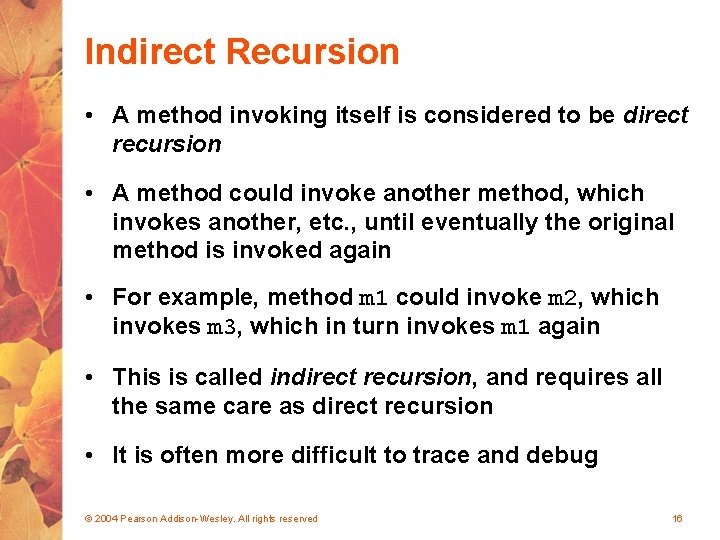Indirect Recursion • A method invoking itself is considered to be direct recursion • A method could invoke another method, which invokes another, etc. , until eventually the original method is invoked again • For example, method m 1 could invoke m 2, which invokes m 3, which in turn invokes m 1 again • This is called indirect recursion, and requires all the same care as direct recursion • It is often more difficult to trace and debug © 2004 Pearson Addison-Wesley. All rights reserved 16Indirect Recursion m 1 m 2 m 3 m 1 m 2 m 1 © 2004 Pearson Addison-Wesley. All rights reserved m 3 m 2 m 3 17Maze Traversal • We can use recursion to find a path through a maze • From each location, we can search in each direction • Recursion keeps track of the path through the maze • The base case is an invalid move or reaching the final destination • See Maze. Search. java (page 583) • See Maze. java (page 584) © 2004 Pearson Addison-Wesley. All rights reserved 19Towers of Hanoi • The Towers of Hanoi is a puzzle made up of three vertical pegs and several disks that slide on the pegs • The disks are of varying size, initially placed on one peg with the largest disk on the bottom with increasingly smaller ones on top • The goal is to move all of the disks from one peg to another under the following rules: § We can move only one disk at a time § We cannot move a larger disk on top of a smaller one © 2004 Pearson Addison-Wesley. All rights reserved 20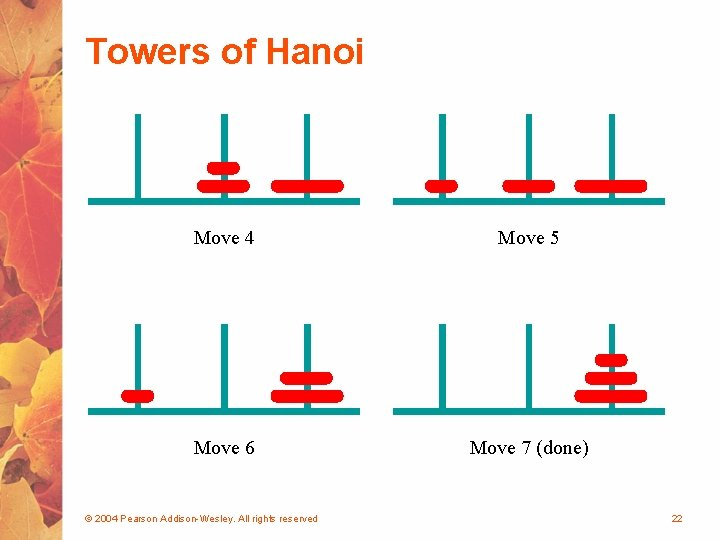Towers of Hanoi • An iterative solution to the Towers of Hanoi is quite complex • A recursive solution is much shorter and more elegant • See Solve. Towers. java (page 590) • See Towers. Of. Hanoi. java (page 591) © 2004 Pearson Addison-Wesley. All rights reserved 23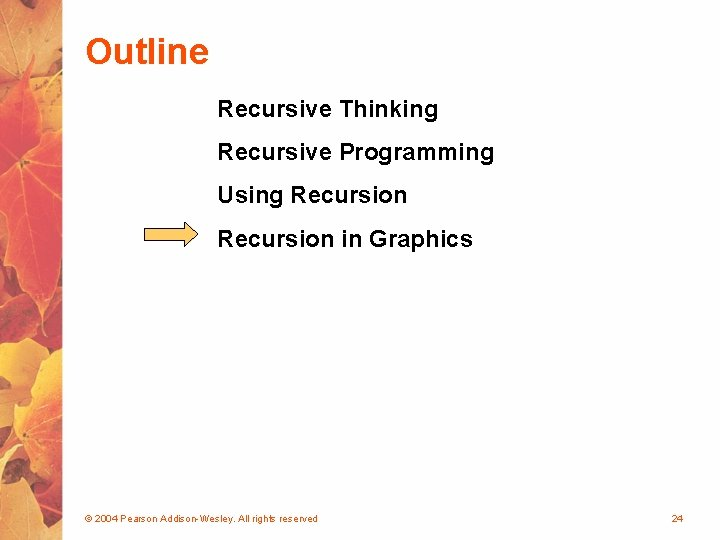Tiled Pictures • Consider the task of repeatedly displaying a set of images in a mosaic § Three quadrants contain individual images § Upper-left quadrant repeats pattern • The base case is reached when the area for the images shrinks to a certain size • See Tiled. Pictures. java (page 594) © 2004 Pearson Addison-Wesley. All rights reserved 25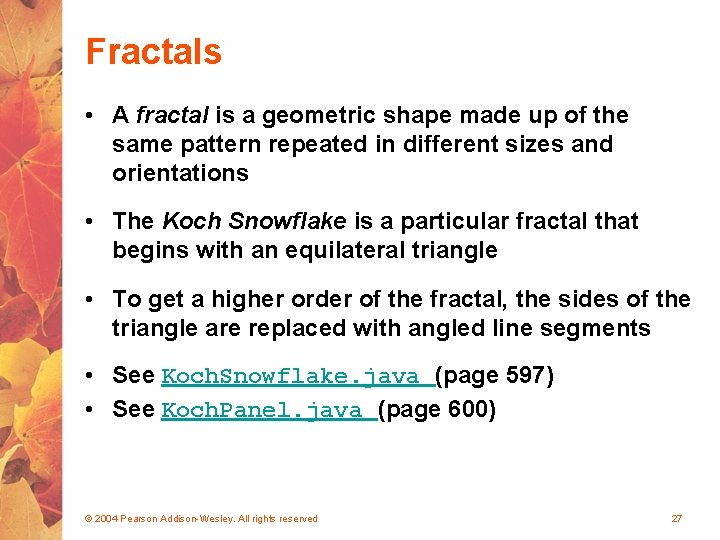Fractals • A fractal is a geometric shape made up of the same pattern repeated in different sizes and orientations • The Koch Snowflake is a particular fractal that begins with an equilateral triangle • To get a higher order of the fractal, the sides of the triangle are replaced with angled line segments • See Koch. Snowflake. java (page 597) • See Koch. Panel. java (page 600) © 2004 Pearson Addison-Wesley. All rights reserved 27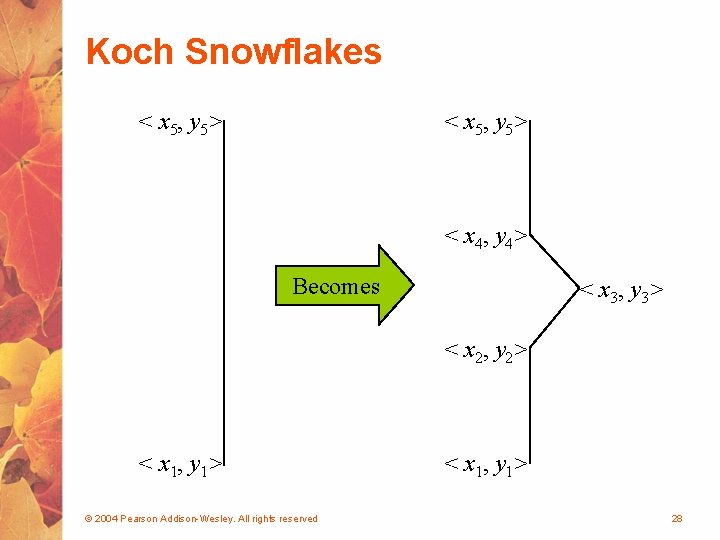Koch Snowflakes < x 5, y 5> < x 4, y 4> Becomes < x 3, y 3> < x 2, y 2> < x 1, y 1> © 2004 Pearson Addison-Wesley. All rights reserved < x 1, y 1> 28